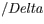# Problem: Use the data given below to construct a Born-Haber cycle to determine the heat of formation of KCl.H °(kJ)K(g) → K+(g) + e-418Cl2(g) → 2 Cl(g)244Cl(g) + e- → Cl-(g)-349KCl(s) → K+(g) + Cl-(g)717

###### FREE Expert Solution

We’re being asked to calculate the enthalpy of formation of KCl, given the lattice energy.

Recall that lattice energy is the energy required to combine two gaseous ions into a solid ionic compound:

Mx+(g) + Ny–(g)  MyNx(s)

To calculate for lattice energy, we need to do the Born-Haber cycle for KCl. We start with the corresponding formation equation for KCl:

K(s) + ½ Cl2(g)  KCl(s), ΔH˚f###### Problem Details

Use the data given below to construct a Born-Haber cycle to determine the heat of formation of KCl.H °(kJ) K(g) → K+(g) + e- 418 Cl2(g) → 2 Cl(g) 244 Cl(g) + e- → Cl-(g) -349 KCl(s) → K+(g) + Cl-(g) 717

What scientific concept do you need to know in order to solve this problem?

Our tutors have indicated that to solve this problem you will need to apply the Born Haber Cycle concept. You can view video lessons to learn Born Haber Cycle. Or if you need more Born Haber Cycle practice, you can also practice Born Haber Cycle practice problems.

What is the difficulty of this problem?

Our tutors rated the difficulty ofUse the data given below to construct a Born-Haber cycle to ...as medium difficulty.

How long does this problem take to solve?

Our expert Chemistry tutor, Sabrina took 10 minutes and 6 seconds to solve this problem. You can follow their steps in the video explanation above.

What professor is this problem relevant for?

Based on our data, we think this problem is relevant for Professor Chen's class at CSULB.